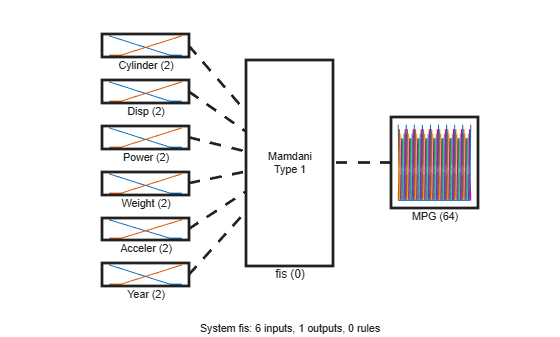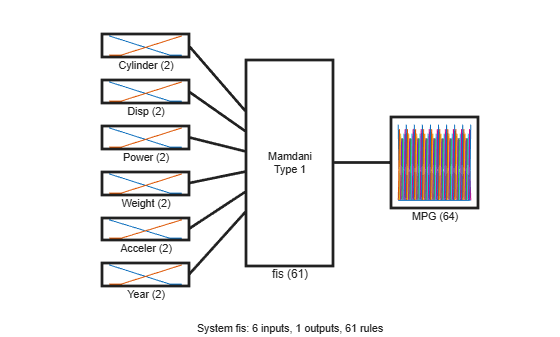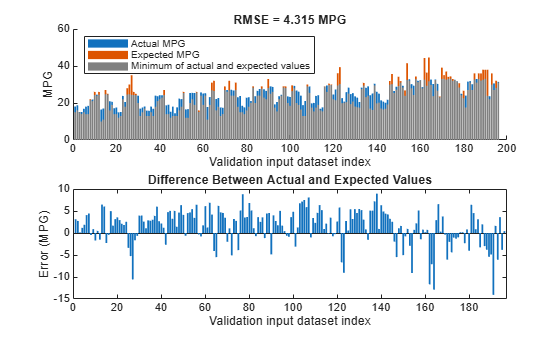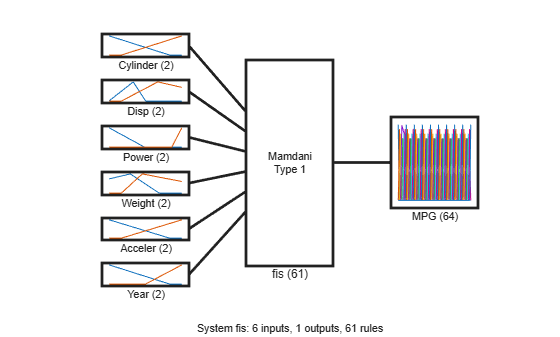Main Content

# Tune Mamdani Fuzzy Inference System

This example shows how to tune membership function (MF) and rule parameters of a Mamdani fuzzy inference system (FIS). This example uses particle swarm and pattern search optimization, which require Global Optimization Toolbox™ software.

Automobile fuel consumption prediction in miles per gallon (MPG) is a typical nonlinear regression problem. It uses several automobile profile attributes to predict fuel consumption. The training data is available in the University of California at Irvine Machine Learning Repository and contains data collected from automobiles of various makes and models.

This example uses the following six input data attributes to predict the output data attribute MPG with a FIS:

1. Number of cylinders

2. Displacement

3. Horsepower

4. Weight

5. Acceleration

6. Model year

### Prepare Data

Load the data. Each row of the dataset obtained from the repository represents a different automobile profile.

`[data,name] = loadGasData;`

Remove leading and trailing whitespace from the attribute names.

`name = strtrim(string(name));`

`data` contains 7 columns, where the first six columns contain the following input attributes.

• Number of cylinders

• Displacement

• Horsepower

• Weight

• Acceleration

• Model year

The seventh column contains the output attribute, MPG.

Create separate input and output data sets, `X` and `Y`, respectively.

```X = data(:,1:6); Y = data(:,7);```

Partition the input and output data sets into training data (odd-indexed samples) and validation data (even-indexed samples).

```trnX = X(1:2:end,:); % Training input data set trnY = Y(1:2:end,:); % Training output data set vldX = X(2:2:end,:); % Validation input data set vldY = Y(2:2:end,:); % Validation output data set```

Extract the range of each data attribute, which you will use for input/output range definition during FIS construction.

`dataRange = [min(data)' max(data)'];`

### Construct FIS using Data Attribute Ranges

Create a Mamdani FIS for tuning.

`fisin = mamfis;`

Add input and output variables to the FIS, where each variable represents one of the data attributes. For each variable, use the corresponding attribute name and range.

To reduce the number of rules, use two MFs for each input variable, which results in ${2}^{6}=64$ input MF combinations. Therefore, the FIS uses a maximum of 64 rules corresponding to the input MF combinations.

To improve data generalization beyond the training data, use 64 MFs for the output variable. Doing so allows the FIS to use a different output MF for each rule.

Both input and output variables use default triangular MFs, which are uniformly distributed over the variable ranges.

```for i = 1:6 fisin = addInput(fisin,dataRange(i,:),'Name',name(i),'NumMFs',2); end fisin = addOutput(fisin,dataRange(7,:),'Name',name(7),'NumMFs',64);```

View the FIS structure. Initially, the FIS has zero rules. The rules of the system are found during the tuning process.

```figure plotfis(fisin)```### Tune FIS with Training Data

Tuning is performed in two steps.

1. Learn the rule base while keeping the input and output MF parameters constant.

2. Tune the parameters of the input/output MFs and rules.

The first step is less computationally expensive due to the small number of rule parameters, and it quickly converges to a fuzzy rule base during training. In the second step, using the rule base from the first step as an initial condition provides fast convergence of the parameter tuning process.

Learn Rules

To learn a rule base, first specify tuning options using a `tunefisOptions` object. Since the FIS allows a large number of output MFs (used in rule consequents), use a global optimization method (genetic algorithm or particle swarm). Such methods perform better in large parameter tuning ranges as compared to local optimization methods (pattern search and simulation annealing). For this example, tune the FIS using the particle swarm optimization method (`'particleswarm'`).

To learn new rules, set the `OptimizationType` to `'learning'`. Restrict the maximum number of rules to 64. The number of tuned rules can be less than this limit, since the tuning process removes duplicate rules.

```options = tunefisOptions('Method','particleswarm',... 'OptimizationType','learning', ... 'NumMaxRules',64);```

If you have Parallel Computing Toolbox™ software, you can improve the speed of the tuning process by setting `options.UseParallel` to `true`. If you do not have Parallel Computing Toolbox software, set `options.UseParallel` to `false`.

Set the maximum number of iterations to 20. To reduce training error in the rule learning process, you can increase the number of iterations. However, using too many iterations can overtune the FIS to the training data, increasing the validation errors.

`options.MethodOptions.MaxIterations = 20;`

Since particle swarm optimization uses random search, to obtain reproducible results, initialize the random number generator to its default configuration.

`rng('default')`

Tune the FIS using the specified tuning data and options.

Learning rules using the `tunefis` function takes approximately 5 minutes. For this example, enable tuning by setting `runtunefis` to `true`. To load pretrained results without running `tunefis`, you can set `runtunefis` to `false`.

`runtunefis = false;`

Parameter settings can be empty when learning new rules. For more information, see `tunefis`.

```if runtunefis fisout1 = tunefis(fisin,[],trnX,trnY,options); %#ok<UNRCH> else tunedfis = load('tunedfismpgprediction.mat'); fisout1 = tunedfis.fisout1; fprintf('Training RMSE = %.3f MPG\n',calculateRMSE(fisout1,trnX,trnY)); end```
```Training RMSE = 4.452 MPG ```

The `Best f(x)` column shows the training root-mean-squared-error (RMSE).

View the structure of the tuned FIS, `fisout1`.

`plotfis(fisout1)`The learning process produces a set of new rules for the FIS. For example, view the descriptions of the first three rules.

`[fisout1.Rules(1:3).Description]' `
```ans = 3x1 string "Cylinder==mf2 & Disp==mf2 & Power==mf2 & Weight==mf2 & Year==mf2 => MPG=mf5 (1)" "Cylinder==mf1 & Power==mf2 & Weight==mf2 & Acceler==mf2 & Year==mf1 => MPG=mf63 (1)" "Cylinder==mf2 & Disp==mf1 & Acceler==mf2 => MPG=mf28 (1)" ```

The learned system should have similar RMSE performance for both the training and validation data sets. To calculate the RMSE for the validation data set, evaluate `fisout1` using validation input data set `vldX`. To hide run-time warnings during evaluation, set all the warning options to `none`.

Calculate the RMSE between the generated output data and the validation output data set `vldY`.

`plotActualAndExpectedResultsWithRMSE(fisout1,vldX,vldY)`Since the training and validation errors are similar, the learned system does not overfit the training data.

Tune All Parameters

After learning the new rules, tune the input/output MF parameters along with the parameters of the learned rules. To obtain the tunable parameters of the FIS, use the `getTunableSettings` function.

`[in,out,rule] = getTunableSettings(fisout1);`

To tune the existing FIS parameter settings without learning new rules, set the `OptimizationType` to `'tuning'`.

`options.OptimizationType = 'tuning';`

Since the FIS already learned rules using the training data, use a local optimization method for fast convergence of the parameter values. For this example, use the pattern search optimization method (`'patternsearch'`).

`options.Method = 'patternsearch';`

Tuning the FIS parameters takes more iterations than the previous rule-learning step. Therefore, increase the maximum number of iterations of the tuning process to 60. As in the first tuning stage, you can reduce training errors by increasing the number of iterations. However, using too many iterations can overtune the parameters to the training data, increasing the validation errors.

`options.MethodOptions.MaxIterations = 60;`

To improve pattern search results, set method option `UseCompletePoll` to true.

`options.MethodOptions.UseCompletePoll = true;`

Tune the FIS parameters using the specified tunable settings, training data, and tuning options.

Tuning parameter values with `tunefis` function takes approximately 5 minutes. To load pretrained results without running `tunefis`, you can set `runtunefis` to `false`.

```if runtunefis rng('default') %#ok<UNRCH> fisout = tunefis(fisout1,[in;out;rule],trnX,trnY,options); else fisout = tunedfis.fisout; fprintf('Training RMSE = %.3f MPG\n',calculateRMSE(fisout,trnX,trnY)); end```
```Training RMSE = 2.903 MPG ```

At the end of the tuning process, some of the tuned MF shapes are different than the original ones.

```figure plotfis(fisout)```### Check Performance

Validate the performance of the tuned FIS, `fisout`, using the validation input data set `vldX`.

Compare the expected MPG obtained from the validation output data set `vldY` and actual MPG generated using `fisout`. Compute the RMSE between these results.

`plotActualAndExpectedResultsWithRMSE(fisout,vldX,vldY);`Tuning the FIS parameters improves the RMSE compared to the results from the initial learned rule base. Since the training and validation errors are similar, the parameters values are not overtuned.

### Conclusion

You can further improve the training error of the tuned FIS by:

• Increasing number of iterations in both the rule-learning and parameter-tuning phases. Doing so increases the duration of the optimization process and can also increase validation error due to overtuned system parameters with the training data.

• Using global optimization methods, such as `ga` and `particleswarm`, in both rule-learning and parameter-tuning phases. `ga` and `particleswarm` perform better for large parameter tuning ranges since they are global optimizers. On the other hand, `patternsearch` and `simulannealbnd` perform better for small parameter ranges since they are local optimizers. If a FIS is generated from training data with `genfis` or a rule base is already added to a FIS using training data, then `patternsearch` and `simulannealbnd` may produce faster convergence as compared to `ga` and `particleswarm`. For more information on these optimization methods and their options, see `ga` (Global Optimization Toolbox), `particleswarm` (Global Optimization Toolbox), `patternsearch` (Global Optimization Toolbox), and `simulannealbnd` (Global Optimization Toolbox).

• Changing the FIS properties, such as the type of FIS, number of inputs, number of input/output MFs, MF types, and number of rules. For fuzzy systems with a large number of inputs, a Sugeno FIS generally converges faster than a Mamdani FIS since a Sugeno system has fewer output MF parameters (if `constant` MFs are used) and faster defuzzification. Small numbers of MFs and rules reduce the number of parameters to tune, producing a faster tuning process. Furthermore, a large number of rules may overfit the training data. In general, for larger fuzzy systems, a FIS tree can produce similar performance with a smaller number of rules as compared to a single FIS. For an example, see Tune FIS Tree for Gas Mileage Prediction.

• Modifying tunable parameter settings for MFs and rules. For example, you can tune the support of a triangular MF without changing its peak location. Doing so reduces the number of tunable parameters and can produce a faster tuning process for specific applications. For rules, you can exclude zero MF indices by setting the `AllowEmpty` tunable setting to `false`, which reduces the overall number of rules during the learning phase.

### Local Functions

```function plotActualAndExpectedResultsWithRMSE(fis,x,y) % Calculate RMSE bewteen actual and expected results [rmse,actY] = calculateRMSE(fis,x,y); % Plot results figure subplot(2,1,1) hold on bar(actY) bar(y) bar(min(actY,y),'FaceColor',[0.5 0.5 0.5]) hold off axis([0 200 0 60]) xlabel("Validation input dataset index"),ylabel("MPG") legend(["Actual MPG" "Expected MPG" "Minimum of actual and expected values"],... 'Location','NorthWest') title("RMSE = " + num2str(rmse) + " MPG") subplot(2,1,2) bar(actY-y) xlabel("Validation input dataset index"),ylabel("Error (MPG)") title("Difference Between Actual and Expected Values") end function [rmse,actY] = calculateRMSE(fis,x,y) % Specify options for FIS evaluation persistent evalOptions if isempty(evalOptions) evalOptions = evalfisOptions("EmptyOutputFuzzySetMessage","none", ... "NoRuleFiredMessage","none","OutOfRangeInputValueMessage","none"); end % Evaluate FIS actY = evalfis(fis,x,evalOptions); % Calculate RMSE del = actY - y; rmse = sqrt(mean(del.^2)); end```

Download now